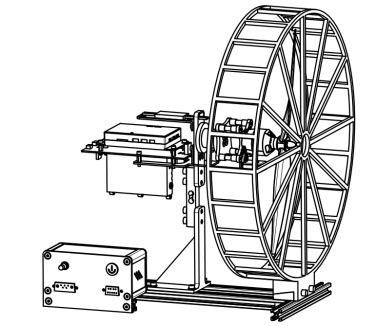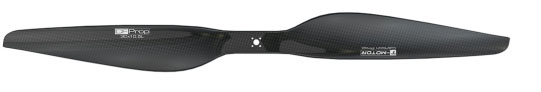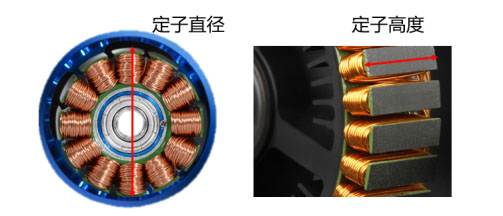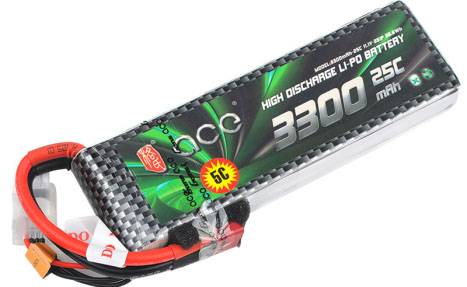Dec. 30, 2021

# Measurement and verification of basic parameters of dynamical systemsCourse Content:

1. Basic parameters of UAV dynamic system

2. UAV power system basic parameter matching principle

3. Measurement of basic parameters of propeller and motor

4. Motor KV value test experiment

5. Current safety test of the dynamic system

Experimental equipment:

WF-EDU-02 UAV dynamic test teaching instrument test kit

Experimental teaching Objectives:

1. Understand propeller, motor, electric modulation, battery model parameters.

2. Master the basic parameter matching principle of UAV dynamic system, and be able to conduct preliminary matching of the dynamic system according to the basic parameters and test results of the dynamic system.

3. Propeller, motor part of the measurement and test verification, deepen the understanding of dynamic system parameters.

I. Explanation of experimental teaching principle:

## 1. Basic parameters of UAV dynamic system

UAV dynamic system is composed of four parts: propeller, motor, electric and battery. The four parts of UAV dynamic system have specific basic parameters. The basic parameters generally include: model, index, structural characteristics and other parameters.

### 1.1 The propeller

Generally speaking, the propeller type (shape) parameters are composed of four digits. The first two digits indicate the diameter of the propeller (in inches, 1 inch ≈25.4mm), and the last two digits indicate the pitch (in inches), also known as the pitch. For example, "1045", "9445", "1812", respectively represent "10" diameter, pitch 4.5 ", "9.4" diameter, pitch 4.5 ", "18" diameter, pitch 12 "propeller.Figure 1.1: Legend of propeller

The pitch in the propeller model parameter is the geometric pitch of the propeller, which refers to the distance advanced by rotating one circle in the non-flowing medium. For example, a propeller has a pitch of 4.5 inches, which means it can travel 4.5 inches in one rotation.

### 1.2 Motor

The common basic parameters of the motor include the motor model, KV value, internal resistance, output shaft diameter, working voltage, maximum current and maximum power, no-load current, and slot pole structure. Taking The Langyu 2212 motor as an example, the basic parameters of the motor are shown in the figure.

 Motor model / Kv value X2212-III/KV980 X2212-III/KV 1250 X2212-III/KV1400 Number of slot poles 12N14P 12N14P 12N14P Interphase internal resistance 92mΩ 54mΩ 43mΩ Motor size the major axis: Ф 27.5*46.0mmStub shaft: Ф 27.5*32.5mm the major axis: Ф 27.5*46.0mmStub shaft: Ф 27.5*32.5mm the major axis: Ф 27.5*46.0mmStub shaft: Ф 27.5*32.5mm Shaft diameter 3.175mm 3.175mm 3.175mm Specification of motor silicone wire 20AWG 100mm 20AWG 100mm 20AWG 100mm Weight of motor （include wire） 57g 59g 57g Weight of motor （exclude wire） / / / Working stable voltage（Lipo) 3-4S 3-4S 3-4S No load current 0.6A/10V 0.8A/10V 1A/10V Maximum continuous power 385W 518W 560W Maximum continuous current 26A/30S 35A/30S 37A/30S Recommended electric regulation 20-30A 30-40A 30-40A Recommended propeller specifications APC9047/9045/8038/1038/1047 APC9047/9060/8060/7060 APC9047/9045/8038/7060/8060

FIG. 1.2.1:2212 Motor parameter table

(1) Structural dimension parameters

The model (appearance) of the brushless motor is composed of four digits, which represent the size of the motor stator. The first two digits represent the stator diameter (unit: mm), and the last two digits represent the stator height (unit: mm). For example, the motor 2212 indicates that the stator diameter is 22mm and the stator height is 12mm. The larger the first two numbers, the larger the diameter, the thicker the motor. The higher the last two numbers, the higher the height, while the higher the motor.FIG. 1.2.2:2212 Motor stator diameter and height

Motor slot pole structure (NP) : N represents the number of slots of the motor, P represents the number of magnetic poles of the motor, for example, "12N14P" represents the number of slots of the motor is 12, the number of magnetic poles is 14, and the magnetic level logarithm is 7 (that is, 7 pairs of magnetic poles).

The number of slots of the motor refers to the number of slots in the stator core of the motor. Since the brushless DC motor is a three-phase motor, the number of slots is a multiple of 3. The number of poles of the motor is the number of magnetic poles on the rotor of the motor. The magnetic poles must be in pairs, so the number of poles is a multiple of 2.Figure 1.2.3: Schematic diagram of motor pole and motor groove

(2) KV value

The KV value of the brushless motor is the parameter representing the motor speed, which refers to the increase of the motor speed (unit r/min, also can be expressed as RPM) when the voltage increases by 1V in the no-load (no propeller installed) state of the motor. For example, "980KV" indicates that the speed increases by 980 r/min for each increase of 1V voltage. Or the speed should be 9800r/min under 10V no-load. (Due to the production quality control problems of the motor and different production batches, the actual KV value of the motor will deviate from the KV value calibrated for the original model, and the deviation is generally within 10%).

The relation between KV value and winding number T:

T, the full English name of Turn, stands for the number of turns of the coil, which means how many times the stator winding coil has been wound. For example, 21T indicates that the stator winding coil has been wound 21 times.

For brushless DC motor of the same size and specification, the size of KV value of the motor can be changed by changing T value.Compared with the same type of motor parameters, the number of turns of the coil is low, the KV value is low, the highest output current is small, the speed is low, the torque is large under the low speed. Coil turns less KV value is high, the highest output current is large, high speed, but the torque is small.

(3) Other parameters

Maximum continuous current (maximum current) and maximum continuous power (maximum power) : The maximum current and maximum power of a brushless motor refers to the maximum current and maximum power that the motor can withstand under normal working conditions. For example, the maximum continuous current "15A/30s" indicates that the motor can work for a maximum of 30 seconds under the current of 15A. Exceeding the time or current will cause the motor to burn out at high temperature.

Operating voltage (maximum number of batteries) : The operating voltage of the brushless motor refers to the voltage value that ensures the normal operation of the motor. In the UAV motor parameter table, the serial number of lithium batteries is usually used to indicate the range of working voltage. For example, working voltage "2s-4S" indicates the working voltage range of 2-4 lithium batteries in series.

### 1.3 Electrically controlled

The parameters of the electric switch are the important basis for selecting the electric switch. Generally, the parameter table of the electric switch manual contains the electric switch model, continuous output current, instantaneous current, BEC, input voltage, weight and volume, as shown in the figure. Continuous output current, instantaneous current, BEC and input voltage are introduced below.Figure 1.3: Electrical tuning legend

(1) Continuous output current

Continuous output current refers to the continuous output current of the ESC in normal working mode, and the unit is A. For example, 30A indicates that the continuous output current of the switch is 30A. Continuous output current is the most important parameter of brushless ESC. Select the matched ESC according to the working current of the motor, otherwise the ESC will burn out.

(2) Instantaneous current

Instantaneous current refers to the maximum current that the current can withstand while operating, and the maximum withstand time is 10s.

(3) Input voltage

The operating voltage range within which the ESC can operate normally.For example, 3-4S LIPO indicates the LIPO battery, 3S or 4S.

(4) the BEC

BEC (Battey Eimination Circuit) is a battery-free Circuit. A Circuit module is set in the ESC, which can supply power to electronic equipment such as receiver and steering gear.For example, 2A@5V indicates that the battery output voltage is converted to 5 V. The maximum output current is 2A.

### 1.4 The batteryFigure 1.4: Grignard cell

The above figure is Li-PO battery commonly used by UAV. The factory standard parameters of the battery mainly include: battery capacity of 3300mAh, maximum discharge rate of 25C, maximum charging rate of 5C, rated voltage (nominal voltage) 11.1V, cell combination mode of 3S1P and battery energy of 35.6Wh.

(1) Capacity (mAh)

The capacity unit of UAV battery is generally expressed in mAh, 1000mAh=1Ah. Take the battery marked 3300mAh as an example, it means that the battery can discharge at 3300mA for one hour.

(2) Charge/discharge ratio (C-rate)

The battery charge/discharge ratio represents the charge/discharge current. Charge/discharge ratio (C) = Charge/discharge current (A)/battery capacity (Ah). For example, battery capacity is 3300mAh, 1C discharge charge/discharge current is 3.3a, sustainable charge/discharge 1 hour; 2C charge/discharge current is 6.6a, can be charged/discharged for 0.5 hours. The maximum discharge rate of the battery is 25C, that is, the maximum discharge current is 82.5a. The maximum charging rate of the battery is 5C, which means the maximum charging current is 16.5a.

Note: In order to prolong the service life of the battery, the general battery should be charged or discharged at a low charge/discharge rate.

(3) Voltage

The rated voltage of the UAV lithium polymer single cell is 3.7V, based on the average operating voltage. The voltage range of the single cell is 2.75-4.2V.When the battery is lower than 2.75V, it will over discharge (over discharge), and when the battery is higher than 4.2V, it will overcharge (overcharge). Generally, the rated voltage or multiple of the rated voltage is rated 3.7V, 7.4V,11.1V,14.8V.

(4) Battery combination

Our common UAV batteries will be labeled 3S1P,6S1P,3S2P, etc., where S(Serial) means battery series, P (Parallel) means battery Parallel. For example, 3S2P refers to that three batteries are connected in series, and then two groups of batteries are connected in parallel to form a battery pack, with a total of six battery cores. Series will increase the battery voltage, single lithium polymer battery cell nominal rated voltage is 3.7V, 3S battery voltage is 3.7x4 =14.8V. Parallel connection increases battery capacity, for example 2600mAh for a single battery and 5200mAh for a 2P battery. There are also batteries that will mark 6cells, which is actually 6S1P connection mode. Because UAV batteries are mostly in series, they are generally referred to as several S batteries, and rarely called several P batteries.

(5) Battery energy (Wh)

Battery energy refers to the stored energy, which is how much work the battery can do in units of Wh. One degree of electricity in life is 1000Wh (1kWh). As you can see from the unit, battery energy and capacity are different concepts. Battery energy (Wh) = battery capacity (mAh) * voltage (V). So when the battery voltage is the same, we can use the battery capacity or battery energy for comparison. When the battery voltage is different, we need to use the battery energy for comparison. Battery energy density refers to the amount of energy a battery can emit per unit mass or volume, reflecting the relationship between battery energy and weight. Energy density (Wh/kg) = battery energy/weight. It can be seen from the formula that the higher the battery energy density is, the smaller the weight is, under the condition that the battery energy remains unchanged. The current common polymer lithium battery energy density is between 110-200Wh/kg.

## 2. Matching principle of basic parameters of motor, ESC and battery:

(1) The principle of consistency of voltage parameter matching, that is, battery voltage, motor working voltage and input voltage parameter matching of ESC should be consistent, all within the range of each other's voltage parameters. For example: the working voltage of the motor is 4-6s, and the input voltage of the ESC is 3-4S. In this case, only 4S battery can be selected for power supply.

(2) The principle of safe matching of current parameters, that is, the continuous output current of ESC should be greater than the maximum current of the motor.

(3) Current safety operation principle, that is, during the operation of the dynamic system, the test current value should be less than the maximum current of the motor. After the motor is matched with the propeller, the dynamic system operation test is carried out. The purpose is to ensure that the tested current value should be less than the maximum allowable current of the motor under 100% throttle state.

E-mail: sandy@wing-flying.com

Add.: 7th Floor, B2#，Animation Building, Sino-Singapore Tianjin Eco-City